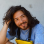# Difference Between Math and Arithmetic

• Updated January 23, 2021
• Pages 3 (562 words)
• Views 221
• Subject
• Category
• Topic
This is FREE sample
This text is free, available online and used for guidance and inspiration. Need a 100% unique paper? Order a custom essay.
• Any subject

You can find so many humanoids among us who have, although they hated it, somehow learned mathematics and arithmetics all through school having absolutely no idea about the unique contrast among the two. You may think there is only a fine line between your least favorite subjects – maths and arithmetic. When you learn that each difference between math and arithmetic are meaningful and are contrasting, you will be shocked to know that this line is really thick!

In this article, we are going to discuss what is the difference between math and Arithmetic that has left so many thinkers thinking!

## Let Us Discuss the Definition of Math

Math is a humongous subject. It has topics that deal with a variety of shapes, many numbers, and various quantities. In this subject, you can find various subcategories that may or may not interest you. They can be Algebra, trigonometry, calculus, or geometry. You always knew arithmetic is a part of math, didn’t you? For those of us who didn’t know it, yes, Arithmetic is a part of math! All these subjects will solve various problems with the help of symbols, formulas, signs, and theorems.

Maths is not only useful when you want to calculate how much a shop vendor is charging you for the good you purchased, but it will also increase your skills at problem-solving and is a great exercise for your brain. In simple words, the definition of math is a subject that explores the relationship that quantities, numbers, and shapes have with one another.

## Uncomplicated Definition of Arithmetic

Arithmetic is one of many unique offshoots found in the ocean called mathematics. When you master Arithmetic, you will know what are the attributes that numbers possess. You will also know how to manipulate them. Arithmetic is all about basic numerical operations around which the mathematical universe revolves. It teaches us how to add numbers; subtract them; multiply them; divide them.

Arithmetic can also sometimes talk about exponents that are a part of multiplication. Arithmetic can also include fractions and percentage that can be regarded as parts of division. In simple words, the definition of arithmetic can be explained as – it is as important to a mathematician as spellings are to a writer.

## Let’s Compare With a Math Vs Arithmetic Comparison Table

To make things easier, we have made a simple table with the main differences between mathematics and arithmetic.

### Basis of comparison Arithmetic Math

• Deals with Arithmetic is all about numbers Math is all about theories
• Operations Addition, subtraction, multiplication, division Trigonometry, calculus, geometry, algebra, arithmetic
• Interchangeable Arithmetic is interchangeable with math Math is not interchangeable with arithmetic

## To Conclude

If you ask any kid they would say that math is the trickiest subject to learn in school. They would also say how vast and difficult it is. However, Arithmetic is simple as it involves only basic operations of mathematics. That’s the main difference between them!

Mathematics is a subject that divides into different pieces just like a tree and involves reading theorems of various great scholars, evidence about them, and theories. Arithmetic can be called as a derivative of mathematics, which explains to us how we can add numbers, subtract them, multiply, and divide them. Every one of us loves Arithmetic for its simplicity. And those of us who don’t, has to love it to make simple, day-to-day calculations.

Cite this paper